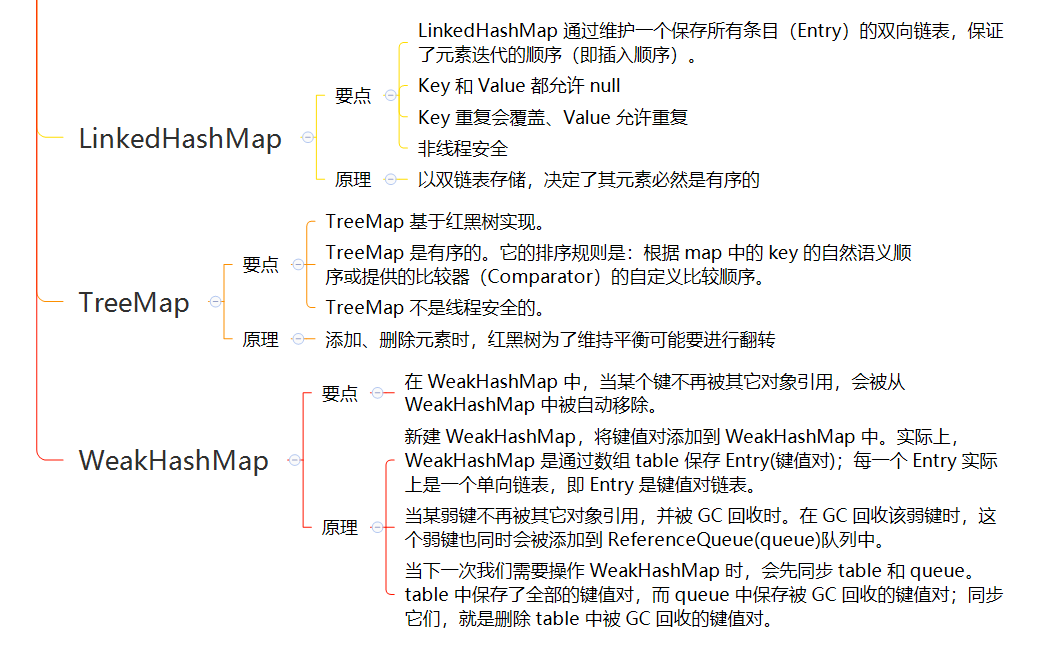# # Java 容器之 Map

📦 本文以及示例源码已归档在 javacore (opens new window)

## # 1. Map 简介

### # 1.1. Map 架构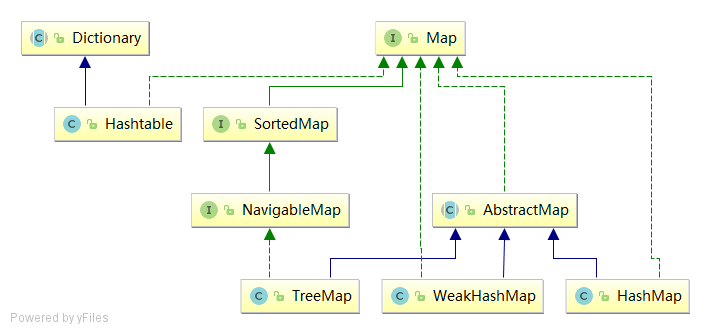Map 家族主要成员功能如下：

• `Map` 是 Map 容器家族的祖先，Map 是一个用于保存键值对(key-value)的接口。Map 中不能包含重复的键；每个键最多只能映射到一个值。
• `AbstractMap` 继承了 `Map` 的抽象类，它实现了 `Map` 中的核心 API。其它 `Map` 的实现类可以通过继承 `AbstractMap` 来减少重复编码。
• `SortedMap` 继承了 `Map` 的接口。`SortedMap` 中的内容是排序的键值对，排序的方法是通过实现比较器(`Comparator`)完成的。
• `NavigableMap` 继承了 `SortedMap` 的接口。相比于 `SortedMap``NavigableMap` 有一系列的“导航”方法；如"获取大于/等于某对象的键值对"、“获取小于/等于某对象的键值对”等等。
• `HashMap` 继承了 `AbstractMap`，但没实现 `NavigableMap` 接口。`HashMap` 的主要作用是储存无序的键值对，而 `Hash` 也体现了它的查找效率很高。`HashMap` 是使用最广泛的 `Map`
• `Hashtable` 虽然没有继承 `AbstractMap`，但它继承了 `Dictionary``Dictionary` 也是键值对的接口），而且也实现 `Map` 接口。因此，`Hashtable` 的主要作用是储存无序的键值对。和 HashMap 相比，`Hashtable` 在它的主要方法中使用 `synchronized` 关键字修饰，来保证线程安全。但是，由于它的锁粒度太大，非常影响读写速度，所以，现代 Java 程序几乎不会使用 `Hashtable` ，如果需要保证线程安全，一般会用 `ConcurrentHashMap` 来替代。
• `TreeMap` 继承了 `AbstractMap`，且实现了 `NavigableMap` 接口。`TreeMap` 的主要作用是储存有序的键值对，排序依据根据元素类型的 `Comparator` 而定。
• `WeakHashMap` 继承了 `AbstractMap``WeakHashMap` 的键是弱引用 （即 `WeakReference`），它的主要作用是当 GC 内存不足时，会自动将 `WeakHashMap` 中的 key 回收，这避免了 `WeakHashMap` 的内存空间无限膨胀。很明显，`WeakHashMap` 适用于作为缓存。

### # 1.2. Map 接口

Map 的定义如下：

``````public interface Map<K,V> { }
``````

Map 是一个用于保存键值对(key-value)的接口。Map 中不能包含重复的键；每个键最多只能映射到一个值。

Map 接口提供三种 `Collection` 视图，允许以键集值集键-值映射关系集的形式访问数据。

Map 有些实现类，可以有序的保存元素，如 `TreeMap`；另一些实现类则不保证顺序，如 `HashMap` 类。

Map 的实现类应该提供 2 个“标准的”构造方法：

• void（无参数）构造方法，用于创建空 Map；
• 带有单个 Map 类型参数的构造方法，用于创建一个与其参数具有相同键-值映射关系的新 Map。

### # 1.3. Map.Entry 接口

`Map.Entry` 一般用于通过迭代器（`Iterator`）访问问 `Map`

`Map.Entry` 是 Map 中内部的一个接口，`Map.Entry` 代表了 键值对 实体，Map 通过 `entrySet()` 获取 `Map.Entry` 集合，从而通过该集合实现对键值对的操作。

### # 1.4. AbstractMap 抽象类

`AbstractMap` 的定义如下：

``````public abstract class AbstractMap<K,V> implements Map<K,V> {}
``````

`AbstractMap` 抽象类提供了 `Map` 接口的核心实现，以最大限度地减少实现 `Map` 接口所需的工作。

### # 1.5. SortedMap 接口

`SortedMap` 的定义如下：

``````public interface SortedMap<K,V> extends Map<K,V> { }
``````

`SortedMap` 继承了 `Map` ，它是一个有序的 `Map`

`SortedMap` 的排序方式有两种：自然排序或者用户指定比较器插入有序 `SortedMap` 的所有元素都必须实现 `Comparable` 接口（或者被指定的比较器所接受）

1. `void`（无参数）构造方法，它创建一个空的有序 `Map`，按照键的自然顺序进行排序。
2. 带有一个 `Comparator` 类型参数的构造方法，它创建一个空的有序 `Map`，根据指定的比较器进行排序。
3. 带有一个 `Map` 类型参数的构造方法，它创建一个新的有序 `Map`，其键-值映射关系与参数相同，按照键的自然顺序进行排序。
4. 带有一个 `SortedMap` 类型参数的构造方法，它创建一个新的有序 `Map`，其键-值映射关系和排序方法与输入的有序 Map 相同。无法保证强制实施此建议，因为接口不能包含构造方法。

### # 1.6. NavigableMap 接口

`NavigableMap` 的定义如下：

``````public interface NavigableMap<K,V> extends SortedMap<K,V> { }
``````

`NavigableMap` 继承了 `SortedMap` ，它提供了丰富的查找方法。

NavigableMap 分别提供了获取“键”、“键-值对”、“键集”、“键-值对集”的相关方法。

`NavigableMap` 提供的功能可以分为 4 类：

• 获取键-值对
• `lowerEntry``floorEntry``ceilingEntry``higherEntry` 方法，它们分别返回与小于、小于等于、大于等于、大于给定键的键关联的 Map.Entry 对象。
• `firstEntry``pollFirstEntry``lastEntry``pollLastEntry` 方法，它们返回和/或移除最小和最大的映射关系（如果存在），否则返回 null。
• 获取键。这个和第 1 类比较类似。
• `lowerKey``floorKey``ceilingKey``higherKey` 方法，它们分别返回与小于、小于等于、大于等于、大于给定键的键。
• 获取键的集合
• `navigableKeySet``descendingKeySet` 分别获取正序/反序的键集。
• 获取键-值对的子集

### # 1.7. Dictionary 抽象类

`Dictionary` 的定义如下：

``````public abstract class Dictionary<K,V> {}
``````

`Dictionary` 是 JDK 1.0 定义的操作键值对的抽象类，它包括了操作键值对的基本方法。

## # 2. HashMap 类

`HashMap` 类是最常用的 `Map`

### # 2.1. HashMap 要点

`HashMap` 的命名，也可以看出：`HashMap` 以散列方式存储键值对

`HashMap` 允许使用空值和空键。（`HashMap` 类大致等同于 `Hashtable`，除了它是不同步的并且允许为空值。）这个类不保序；特别是，它的元素顺序可能会随着时间的推移变化。

`HashMap` 有两个影响其性能的参数：初始容量和负载因子

• 容量是哈希表中桶的数量，初始容量就是哈希表创建时的容量。
• 加载因子是散列表在其容量自动扩容之前被允许的最大饱和量。当哈希表中的 entry 数量超过负载因子和当前容量的乘积时，散列表就会被重新映射（即重建内部数据结构），一般散列表大约是存储桶数量的两倍。

`HashMap` 不是线程安全的。

### # 2.2. HashMap 原理

#### # HashMap 数据结构

`HashMap` 的核心字段：

• `table` - `HashMap` 使用一个 `Node<K,V>[]` 类型的数组 `table` 来储存元素。
• `size` - 初始容量。 初始为 16，每次容量不够自动扩容
• `loadFactor` - 负载因子。自动扩容之前被允许的最大饱和量，默认 0.75。
``````public class HashMap<K,V> extends AbstractMap<K,V>
implements Map<K,V>, Cloneable, Serializable {

// 该表在初次使用时初始化，并根据需要调整大小。分配时，长度总是2的幂。
transient Node<K,V>[] table;
// 保存缓存的 entrySet()。请注意，AbstractMap 字段用于 keySet() 和 values()。
transient Set<Map.Entry<K,V>> entrySet;
// map 中的键值对数
transient int size;
// 这个HashMap被结构修改的次数结构修改是那些改变HashMap中的映射数量或者修改其内部结构（例如，重新散列）的修改。
transient int modCount;
// 下一个调整大小的值（容量*加载因子）。
int threshold;
// 散列表的加载因子
}
``````

#### # HashMap 构造方法

``````public HashMap(); // 默认加载因子0.75
public HashMap(int initialCapacity); // 默认加载因子0.75；以 initialCapacity 初始化容量
public HashMap(Map<? extends K, ? extends V> m) // 默认加载因子0.75
``````

#### # put 方法的实现

put 方法大致的思路为：

• 对 key 的 `hashCode()` 做 hash 计算，然后根据 hash 值再计算 Node 的存储位置;
• 如果没有哈希碰撞，直接放到桶里；如果有哈希碰撞，以链表的形式存在桶后。
• 如果哈希碰撞导致链表过长(大于等于 `TREEIFY_THRESHOLD`，数值为 8)，就把链表转换成红黑树；
• 如果节点已经存在就替换旧值
• 桶数量超过容量*负载因子（即 load factor * current capacity），HashMap 调用 `resize` 自动扩容一倍

``````public V put(K key, V value) {
return putVal(hash(key), key, value, false, true);
}

// hashcode 无符号位移 16 位
static final int hash(Object key) {
int h;
return (key == null) ? 0 : (h = key.hashCode()) ^ (h >>> 16);
}

final V putVal(int hash, K key, V value, boolean onlyIfAbsent,
boolean evict) {
Node<K,V>[] tab; Node<K,V> p; int n, i;
// tab 为空则创建
if ((tab = table) == null || (n = tab.length) == 0)
n = (tab = resize()).length;
// 计算 index，并对 null 做处理
if ((p = tab[i = (n - 1) & hash]) == null)
tab[i] = newNode(hash, key, value, null);
else {
Node<K,V> e; K k;
// 节点存在
if (p.hash == hash &&
((k = p.key) == key || (key != null && key.equals(k))))
e = p;
// 该链为树
else if (p instanceof TreeNode)
e = ((TreeNode<K,V>)p).putTreeVal(this, tab, hash, key, value);
// 该链为链表
else {
for (int binCount = 0; ; ++binCount) {
if ((e = p.next) == null) {
p.next = newNode(hash, key, value, null);
if (binCount >= TREEIFY_THRESHOLD - 1) // -1 for 1st
treeifyBin(tab, hash);
break;
}
if (e.hash == hash &&
((k = e.key) == key || (key != null && key.equals(k))))
break;
p = e;
}
}
// 写入
if (e != null) { // existing mapping for key
V oldValue = e.value;
if (!onlyIfAbsent || oldValue == null)
e.value = value;
afterNodeAccess(e);
return oldValue;
}
}
++modCount;
if (++size > threshold)
resize();
afterNodeInsertion(evict);
return null;
}
``````

#### # get 方法的实现

• 对 key 的 hashCode() 做 hash 计算，然后根据 hash 值再计算桶的 index

• 如果桶中的第一个节点命中，直接返回；

• 如果有冲突，则通过 `key.equals(k)` 去查找对应的 entry

• 若为树，则在红黑树中通过 key.equals(k) 查找，O(logn)；

• 若为链表，则在链表中通过 key.equals(k) 查找，O(n)。

``````public V get(Object key) {
Node<K,V> e;
return (e = getNode(hash(key), key)) == null ? null : e.value;
}

final Node<K,V> getNode(int hash, Object key) {
Node<K,V>[] tab; Node<K,V> first, e; int n; K k;
if ((tab = table) != null && (n = tab.length) > 0 &&
(first = tab[(n - 1) & hash]) != null) {
// 直接命中
if (first.hash == hash && // always check first node
((k = first.key) == key || (key != null && key.equals(k))))
return first;
// 未命中
if ((e = first.next) != null) {
// 在树中 get
if (first instanceof TreeNode)
return ((TreeNode<K,V>)first).getTreeNode(hash, key);
// 在链表中 get
do {
if (e.hash == hash &&
((k = e.key) == key || (key != null && key.equals(k))))
return e;
} while ((e = e.next) != null);
}
}
return null;
}
``````

#### # hash 方法的实现

HashMap 计算桶下标（index）公式：`key.hashCode() ^ (h >>> 16)`

`get``put` 的过程中，计算下标时，先对 `hashCode` 进行 `hash` 操作，然后再通过 `hash` 值进一步计算下标，如下图所示：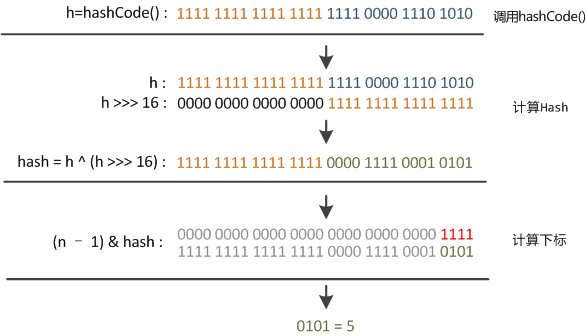``````static final int hash(Object key) {
int h;
return (key == null) ? 0 : (h = key.hashCode()) ^ (h >>> 16);
}
``````

``````(n - 1) & hash
``````

Improve the performance of java.util.HashMap under high hash-collision conditions by using balanced trees rather than linked lists to store map entries. Implement the same improvement in the LinkedHashMap class.

1. 首先根据 hashCode()做 hash，然后确定 bucket 的 index；

2. 如果 bucket 的节点的 key 不是我们需要的，则通过 keys.equals()在链中找。

#### # resize 的实现

`put` 时，如果发现目前的 bucket 占用程度已经超过了 Load Factor 所希望的比例，那么就会发生 resize。在 resize 的过程，简单的说就是把 bucket 扩充为 2 倍，之后重新计算 index，把节点再放到新的 bucket 中。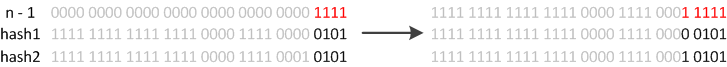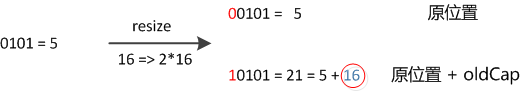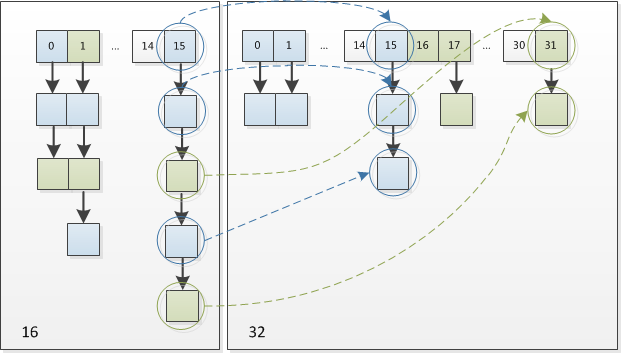``````final Node<K,V>[] resize() {
Node<K,V>[] oldTab = table;
int oldCap = (oldTab == null) ? 0 : oldTab.length;
int oldThr = threshold;
int newCap, newThr = 0;
if (oldCap > 0) {
// 超过最大值就不再扩充了，就只好随你碰撞去吧
if (oldCap >= MAXIMUM_CAPACITY) {
threshold = Integer.MAX_VALUE;
return oldTab;
}
// 没超过最大值，就扩充为原来的 2 倍
else if ((newCap = oldCap << 1) < MAXIMUM_CAPACITY &&
oldCap >= DEFAULT_INITIAL_CAPACITY)
newThr = oldThr << 1; // double threshold
}
else if (oldThr > 0) // initial capacity was placed in threshold
newCap = oldThr;
else {               // zero initial threshold signifies using defaults
newCap = DEFAULT_INITIAL_CAPACITY;
}

// 计算新的 resize 上限
if (newThr == 0) {
float ft = (float)newCap * loadFactor;
newThr = (newCap < MAXIMUM_CAPACITY && ft < (float)MAXIMUM_CAPACITY ?
(int)ft : Integer.MAX_VALUE);
}
threshold = newThr;
@SuppressWarnings({"rawtypes","unchecked"})
Node<K,V>[] newTab = (Node<K,V>[])new Node[newCap];
table = newTab;
if (oldTab != null) {
// 把每个 bucket 都移动到新的 buckets 中
for (int j = 0; j < oldCap; ++j) {
Node<K,V> e;
if ((e = oldTab[j]) != null) {
oldTab[j] = null;
if (e.next == null)
newTab[e.hash & (newCap - 1)] = e;
else if (e instanceof TreeNode)
((TreeNode<K,V>)e).split(this, newTab, j, oldCap);
else { // preserve order
Node<K,V> loHead = null, loTail = null;
Node<K,V> hiHead = null, hiTail = null;
Node<K,V> next;
do {
next = e.next;
// 原索引
if ((e.hash & oldCap) == 0) {
if (loTail == null)
else
loTail.next = e;
loTail = e;
}
// 原索引+oldCap
else {
if (hiTail == null)
else
hiTail.next = e;
hiTail = e;
}
} while ((e = next) != null);
// 原索引放到bucket里
if (loTail != null) {
loTail.next = null;
}
// 原索引+oldCap放到bucket里
if (hiTail != null) {
hiTail.next = null;
}
}
}
}
}
return newTab;
}
``````

`LinkedHashMap` 通过维护一个保存所有条目（Entry）的双向链表，保证了元素迭代的顺序（即插入顺序）

`LinkedHashMap` 通过维护一对 `LinkedHashMap.Entry<K,V>` 类型的头尾指针，以双链表形式，保存所有数据

``````public class LinkedHashMap<K,V>
extends HashMap<K,V>
implements Map<K,V> {

// 双链表的头指针
// 双链表的尾指针
// 迭代排序方法：true 表示访问顺序；false 表示插入顺序
final boolean accessOrder;
}
``````

`LinkedHashMap` 继承了 `HashMap``put` 方法，本身没有实现 `put` 方法。

## # 4. TreeMap 类

### # 4.1. TreeMap 要点

`TreeMap` 基于红黑树实现。

`TreeMap` 是有序的。它的排序规则是：根据 map 中的 key 的自然语义顺序或提供的比较器（`Comparator`）的自定义比较顺序。

TreeMap 不是线程安全的。

### # 4.2. TreeMap 原理

#### # put 方法

``````public V put(K key, V value) {
Entry<K,V> t = root;
// 如果根节点为 null，插入第一个节点
if (t == null) {
compare(key, key); // type (and possibly null) check

root = new Entry<>(key, value, null);
size = 1;
modCount++;
return null;
}
int cmp;
Entry<K,V> parent;
// split comparator and comparable paths
Comparator<? super K> cpr = comparator;
// 每个节点的左孩子节点的值小于它；右孩子节点的值大于它
// 如果有比较器，使用比较器进行比较
if (cpr != null) {
do {
parent = t;
cmp = cpr.compare(key, t.key);
if (cmp < 0)
t = t.left;
else if (cmp > 0)
t = t.right;
else
return t.setValue(value);
} while (t != null);
}
// 没有比较器，使用 key 的自然顺序进行比较
else {
if (key == null)
throw new NullPointerException();
@SuppressWarnings("unchecked")
Comparable<? super K> k = (Comparable<? super K>) key;
do {
parent = t;
cmp = k.compareTo(t.key);
if (cmp < 0)
t = t.left;
else if (cmp > 0)
t = t.right;
else
return t.setValue(value);
} while (t != null);
}
// 通过上面的遍历未找到 key 值，则新插入节点
Entry<K,V> e = new Entry<>(key, value, parent);
if (cmp < 0)
parent.left = e;
else
parent.right = e;
// 插入后，为了维持红黑树的平衡需要调整
fixAfterInsertion(e);
size++;
modCount++;
return null;
}
``````

#### # get 方法

``````public V get(Object key) {
Entry<K,V> p = getEntry(key);
return (p==null ? null : p.value);
}

final Entry<K,V> getEntry(Object key) {
// Offload comparator-based version for sake of performance
if (comparator != null)
return getEntryUsingComparator(key);
if (key == null)
throw new NullPointerException();
@SuppressWarnings("unchecked")
Comparable<? super K> k = (Comparable<? super K>) key;
Entry<K,V> p = root;
// 按照二叉树搜索的方式进行搜索，搜到返回
while (p != null) {
int cmp = k.compareTo(p.key);
if (cmp < 0)
p = p.left;
else if (cmp > 0)
p = p.right;
else
return p;
}
return null;
}
``````

### # 4.3. remove 方法

``````public V remove(Object key) {
Entry<K,V> p = getEntry(key);
if (p == null)
return null;

V oldValue = p.value;
deleteEntry(p);
return oldValue;
}
private void deleteEntry(Entry<K,V> p) {
modCount++;
size--;

// 如果当前节点有左右孩子节点，使用后继节点替换要删除的节点
// If strictly internal, copy successor's element to p and then make p
// point to successor.
if (p.left != null && p.right != null) {
Entry<K,V> s = successor(p);
p.key = s.key;
p.value = s.value;
p = s;
} // p has 2 children

// Start fixup at replacement node, if it exists.
Entry<K,V> replacement = (p.left != null ? p.left : p.right);

if (replacement != null) { // 要删除的节点有一个孩子节点
replacement.parent = p.parent;
if (p.parent == null)
root = replacement;
else if (p == p.parent.left)
p.parent.left  = replacement;
else
D:\codes\zp\java\database\docs\redis\分布式锁.md           p.parent.right = replacement;

// Null out links so they are OK to use by fixAfterDeletion.
p.left = p.right = p.parent = null;

// Fix replacement
if (p.color == BLACK)
fixAfterDeletion(replacement);
} else if (p.parent == null) { // return if we are the only node.
root = null;
} else { //  No children. Use self as phantom replacement and unlink.
if (p.color == BLACK)
fixAfterDeletion(p);

if (p.parent != null) {
if (p == p.parent.left)
p.parent.left = null;
else if (p == p.parent.right)
p.parent.right = null;
p.parent = null;
}
}
}
``````

### # 4.4. TreeMap 示例

``````public class TreeMapDemo {

private static final String[] chars = "A B C D E F G H I J K L M N O P Q R S T U V W X Y Z".split(" ");

public static void main(String[] args) {
TreeMap<Integer, String> treeMap = new TreeMap<>();
for (int i = 0; i < chars.length; i++) {
treeMap.put(i, chars[i]);
}
System.out.println(treeMap);
Integer low = treeMap.firstKey();
Integer high = treeMap.lastKey();
System.out.println(low);
System.out.println(high);
Iterator<Integer> it = treeMap.keySet().iterator();
for (int i = 0; i <= 6; i++) {
if (i == 3) { low = it.next(); }
if (i == 6) { high = it.next(); } else { it.next(); }
}
System.out.println(low);
System.out.println(high);
System.out.println(treeMap.subMap(low, high));
System.out.println(treeMap.tailMap(low));
}
}
``````

## # 5. WeakHashMap

WeakHashMap 的定义如下：

``````public class WeakHashMap<K,V>
extends AbstractMap<K,V>
implements Map<K,V> {}
``````

WeakHashMap 继承了 AbstractMap，实现了 Map 接口。

WeakHashMap 的 key 是弱键，即是 WeakReference 类型的；ReferenceQueue 是一个队列，它会保存被 GC 回收的弱键。实现步骤是：

1. 新建 WeakHashMap，将键值对添加到 WeakHashMap 中。实际上，WeakHashMap 是通过数组 table 保存 Entry(键值对)；每一个 Entry 实际上是一个单向链表，即 Entry 是键值对链表。
2. 当某弱键不再被其它对象引用，并被 GC 回收时。在 GC 回收该弱键时，这个弱键也同时会被添加到 ReferenceQueue(queue)队列中。
3. 当下一次我们需要操作 WeakHashMap 时，会先同步 table 和 queue。table 中保存了全部的键值对，而 queue 中保存被 GC 回收的键值对；同步它们，就是删除 table 中被 GC 回收的键值对。

## # 6. 总结

### # 6.1. Map 简介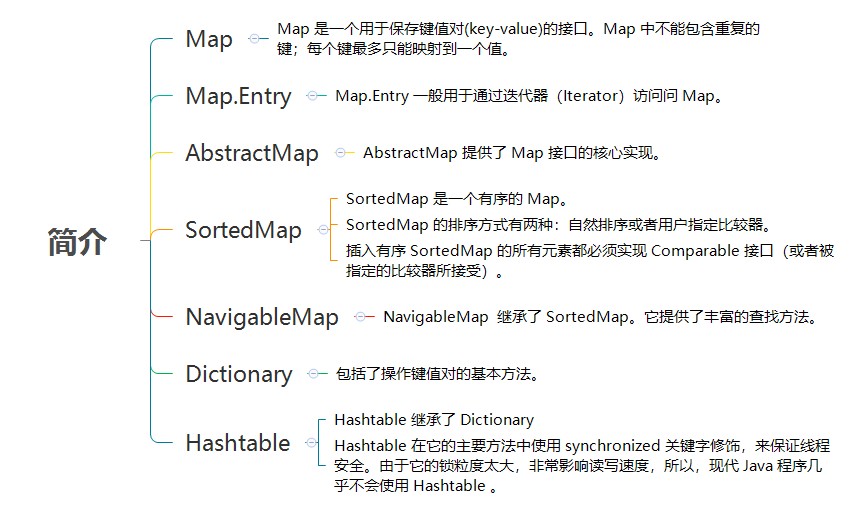### # 6.2. HashMap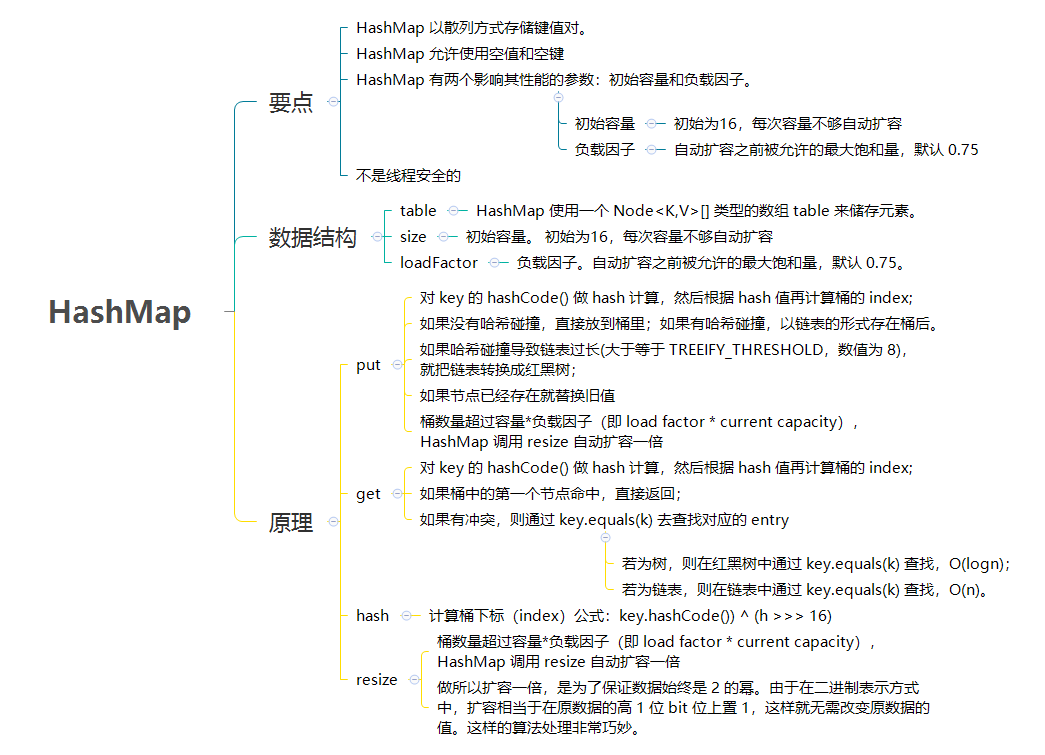### # 6.3. 其他 Map